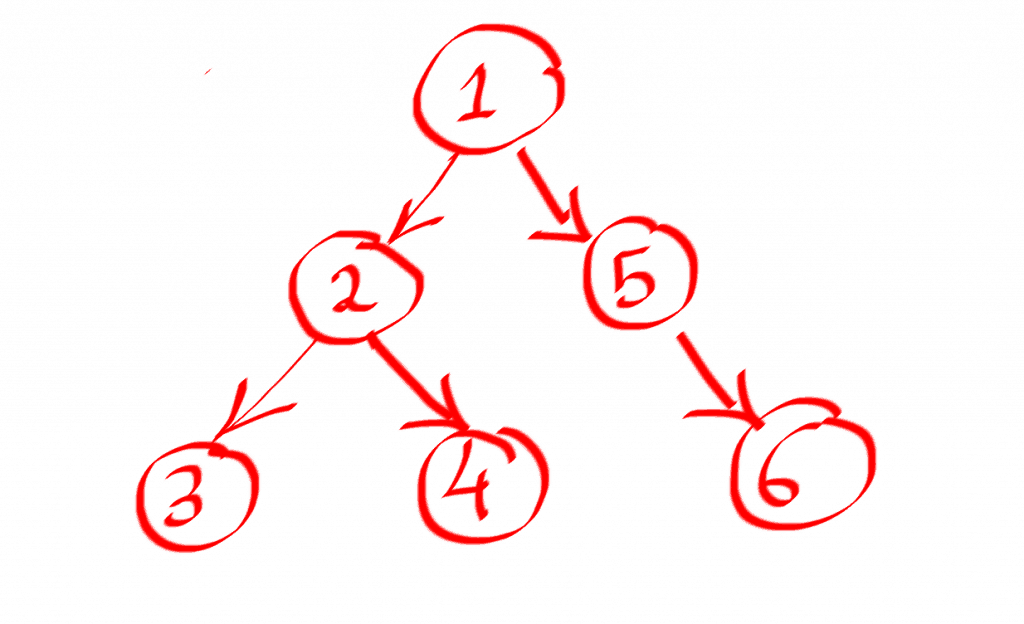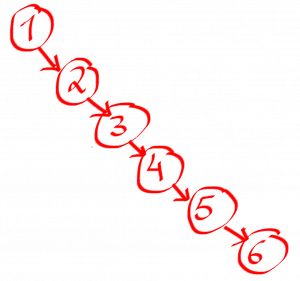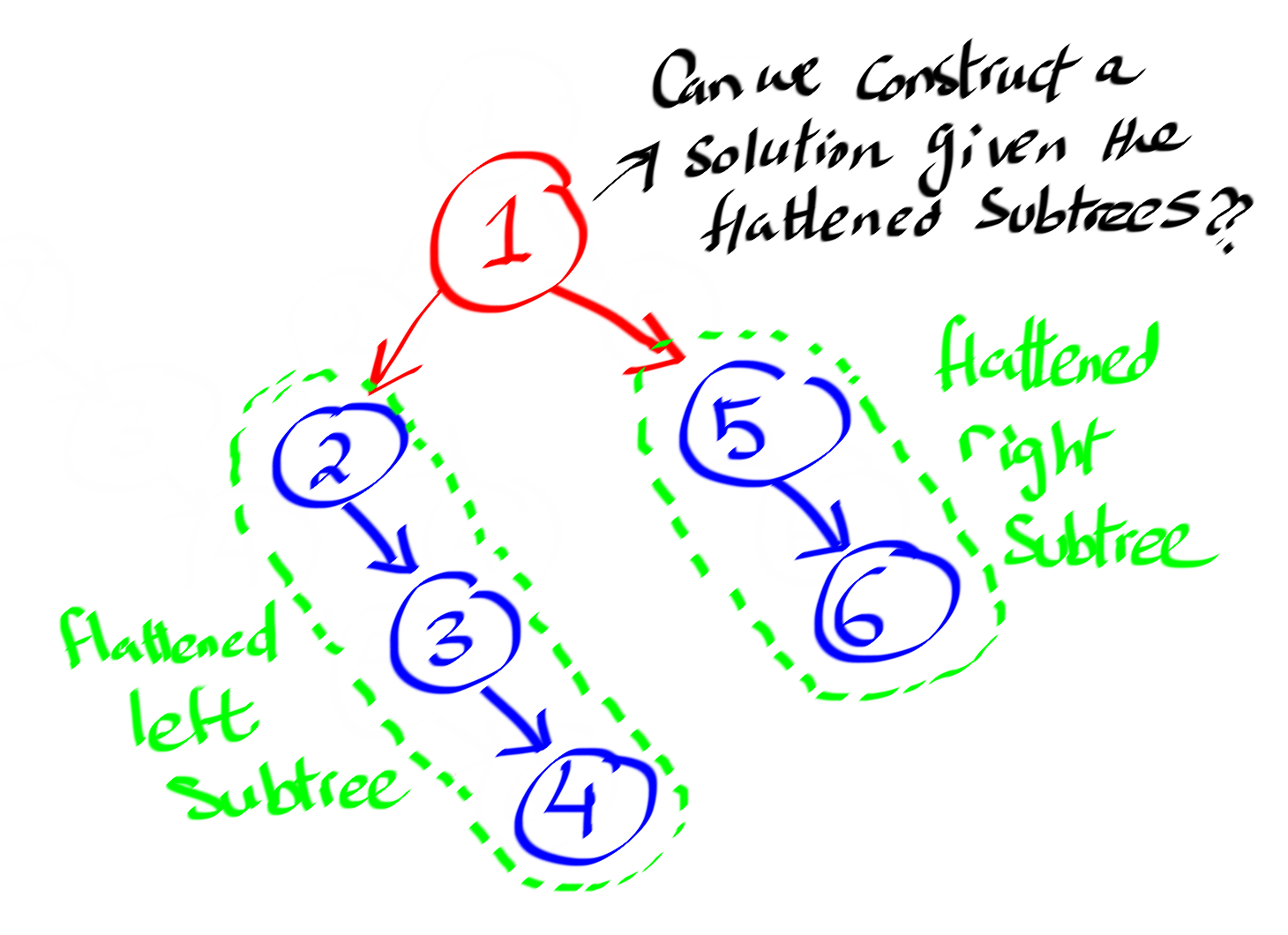In this article, I will solve a common Tree data-structure question. This question also appears frequently in coding interviews.

In solving this question, I will also teach you the thought process that I go through when I encounter problems like this one so you can solve similar problems on your own if you encounter them.

I am also going to provide you with an in-depth explanation of the code, how it works, and what its time complexity is.

## The Question

The question goes as follows:

Given a binary tree, flatten the tree into a linked list in place.

For example, if this is the input treeThe output linked list should look like thisAnd since we are making this conversion in place, you can assume that the Tree’s right pointer is equivalent to a Linked List’s next pointer.

## The Thought Process

As with all tree data structure questions, the first thing that comes to my mind when I am faced with a tree data-structure problem is to think if there is a way to solve the problem recursively through a divide and conquer algorithm.

In other words,  can I construct a solution to the main problem given the solutions of subproblems?

Pro tip: In most tree data-structure questions, this is actually true.

Alright, let’s go back to our problem and try to examine it again from this angle.

By looking at the example shown, it is very obvious that the head of the output linked list is the root of the tree node, followed by a flattened left subtree, which is followed by a flattened right subtree.

This simple description above is our solution.

Well, flattening the left subtree and the right subtree are the sub-problems that I talked about earlier which we can just delegate to the magic of recursion.

Now the question is, given the solutions of these subproblems, how can we construct a solution to the main tree.

Let’s first see how the tree will look like after we flatten the left and right subtrees.

If we recursively flatten the left subtree and the right subtree, we will not precisely end up with the output linked list that we are looking for.

Instead, we will end up with a tree that looks like this.This means we still need to do some more work to construct the final output, but we are in a much better state now.

So how can we do that?

It’s actually very simple.

Step 1: The right pointer of root should point to the head of the flattened left subtree

Step 2: The right pointer of the tail of the left subtree should point to the head of the right subtree

Step 3: The left pointer of root should point to None

## The Code

Now after this thought process that we went through, translating the solution that we came up with into code is very straight-forward.

That’s why I encourage you, whether you are in a coding interview or not, to spend some time thinking about your solution and improving it before you dive into writing code.

Because most of the time, writing the code is the easiest part.

Now let’s start coding our solution up

``````def flatten(root):
if root is not None:
flatten(root.left)
flatten(root.right)
if root.left is not None:
current = root.left
while current.right is not None:
current = current.right

current.right = root.right
root.right = root.left
root.left = None``````

## Explaining the Code

Since this is a recursive solution, you have to think of the base case(s).

Otherwise your code will run infinitely and neither your processor or your memory will be happy :).

Personally, when I am solving a problem in a recursive manner, I tend to think about the recursive part of the solution first before I start thinking about the base cases.

But it’s up to you to figure out which way you’re more comfortable with.

### So what do you think the base case is for this problem?

To answer this, let’s first remember that our function doesn’t actually return anything. Everything happens in place.

Since we are recursively flattening the left and right subtrees for each node, the questions is when do we stop?

We can stop when we encounter a None tree node.

When we encounter a None tree node, we call it a day and we stop recursing any further.

So the base case is:

``if root is None: return``

But instead of saying that, we can also also embed all your logic in the opposite if statement

``if root is not None: # your logic here``

And this is what we have in line number 2

Line number 3 and line number 4 are the magical recursive function calls that solve the subproblems for the left and right subtrees.

``````flatten(root.left)
flatten(root.right)``````

Starting from line number 5, what we are essentially doing is constructing the solution of the main problem from the solutions of subproblems.

But first I want you notice something.

What do you think should happen if our tree doesn’t have a left subtree?

As a matter of fact, if there is no left subtree then we don’t need to do any extra work since the right subtree is already flattened, the root’s left pointer is pointing to None, and the root’s right pointer is pointing to the head of the right subtree.

Sweet! we are done.

It’s only if the left subtree exists that we need to do some extra work.

Specifically, we need to do 3 things:

### 1. Find the tail of the flattened left subtree

Since the left subtree is already flattened, you can use a simple loop to reach the tail

``````current = root.left
while current.right is not None:
current = current.right``````

After the loop terminates, current will be pointing to the tail of the left subtree.

### 2. Connect the tail of the left subtree with the head of the flattened right subtree

Now that we identified the tail of the left subtree, we need to modify the tail’s right(next) pointer to point to the head of the flattened right subtree.

This is what this pice of code does.

``current.right = root.right``

### 3. Modify the root’s left and right pointers

Finally, we need to modify the root’s right pointer to point to the flattened left subtree and left one to point to None.

``````root.right = root.left
root.left = None``````

## Time Complexity

We can use Master theorem to find out the time complexity for our algorithm.

If you are not familiar with Master theorem, I highly recommend that you take a look and familiarize yourself with it.

Sometimes, using Master theorem is the simplest way to analyze the time complexity of a recursive algorithm.

Now let’s see how we can analyze our recursive algorithm.

Basically, to solve this problem for a tree of size n, we need to solve two subproblems for the left and right subtrees.

In the average case, let’s assume that each subtree will be of size (n/2).

After the two subproblems have been resolved, constructing the solution of the original problem requires finding the tail of the left subtree which is O(log n).

With this information, we can write the recursive formula as:

T(n) = 2 * T(n / 2) + o(log n)

By applying the Master theorem to this formula, we get that the run time is O(n).

## Struggling with Tree data structure questions?

### Master Tree coding interview questions with my e-book

Here is what you will get in the e-book:

• 14 problems that cover the majority of ideas for Tree coding interview questions